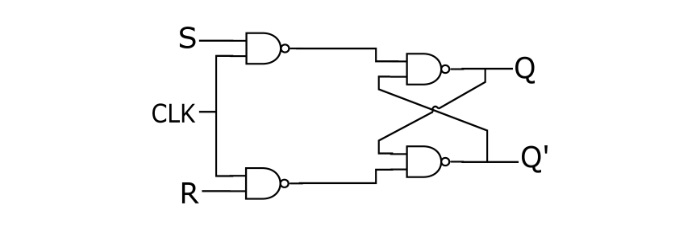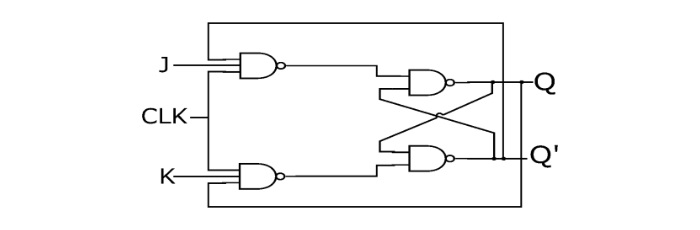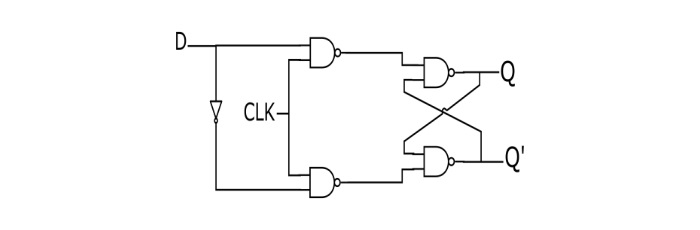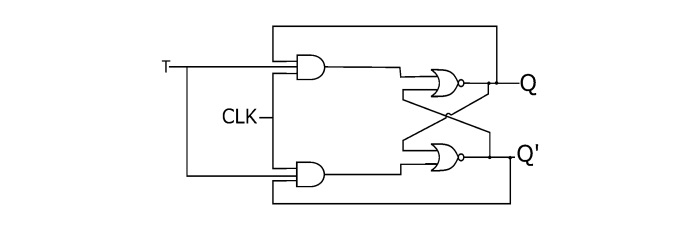# Digital Electronics Flip-flops and their Types

A flip-flop is a sequential digital electronic circuit having two stable states that can be used to store one bit of binary data. Flip-flops are the fundamental building blocks of all memory devices.

## Types of Flip–Flops

• S-R flip-flop
• J-K flip-flop
• D flip-flop
• T flip-flop

## S-R Flip-flop

• This is the simplest flip-flop circuit. It has a set input (S) and a reset input (R). When in this circuit when S is set as active, the output Q would be high and the Q’ will be low. If R is set to active then the output Q is low and the Q’ is high. Once the outputs are established, the results of the circuit are maintained until S or R get changed, or the power is turned off.• Truth table of S-R flip-flop
SRQState
000No Change
010Reset
101Set
11X
• Characteristics Table of S-R flip-flop
SRQ(t)Q(t+1)
0000
0011
0100
0110
1001
1011
110X
111X
• Characteristics equation of S-R flip-flop

$$\mathrm{Q(t+1)=S+RQ(t)}$$

## J-K Flip-flop

• Because of the invalid state corresponding to S=R=1 in the SR flip-flop, there is a need of another flip-flop. The JK flip-flop operates with only positive or negative clock transitions. The operation of the JK flip-flop is similar to the SR flip-flop. When the input J and K are different then the output Q takes the value of J at the next clock edge. When J and K both are low then NO change occurs at the output. If both J and K are high, then at the clock edge, the output will toggle from one state to the other.• Truth table of JK flip-flop
JKQState
000No Change
010Reset
101Set
11TogglesToggle
• Characteristics table of JK flip-flop
JKQ(t)Q(t+1)
0000
0011
0100
0110
1001
1011
1101
1110
• Characteristics equation of JK flip-flop

$$\mathrm{Q(t+1)=jkQ(t)+K`Q(t)}$$

## D Flip-flop

• In a D flip-flop, the output can only be changed at positive or negative clock transitions, and when the inputs changed at other times, the output will remain unaffected. The D flip-flops are generally used for shift-registers and counters. The change in output state of D flip-flop depends upon the active transition of clock. The output (Q) is same as input and changes only at active transition of clock• Truth table of D flip-flop
DQ
00
11
• Characteristics equation of D flip-flop

$$\mathrm{Q(t+1)=D}$$

## T Flip-flop

• A T flip-flop (Toggle Flip-flop) is a simplified version of JK flip-flop. The T flop is obtained by connecting the J and K inputs together. The flip-flop has one input terminal and clock input. These flip-flops are said to be T flip-flops because of their ability to toggle the input state. Toggle flip-flops are mostly used in counters.• Truth Table of T flip-flop
TQ(t)Q(t+1)
000
011
101
110
• Characteristics equation of T flip-flop

$$\mathrm{Q(t+1)=T'Q(t)+TQ(t)'=T\oplus Q(t)}$$

## Applications of Flip-flops

• Counters
• Shift Registers
• Storage Registers, etc.